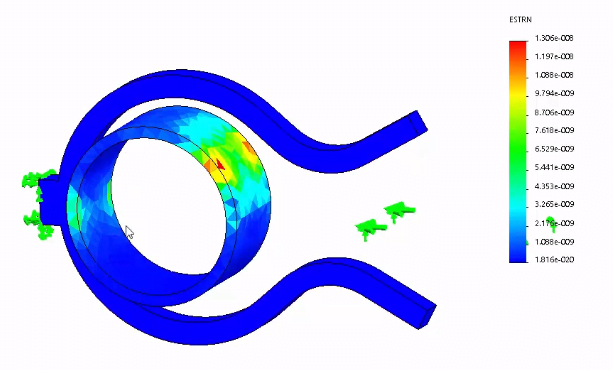Select Page

The concept of nonlinear

Linear static analysis assumes that the relationship between loads and the induced response is linear. For example, if you double the magnitude of loads, the response (displacements, strains, stresses, reaction forces, etc.), will also double.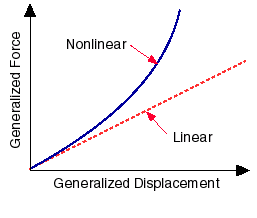Pressure drop analysis using the air flow simulation

All real structures behave nonlinearly in one way or another at some level of loading. In many other cases, the linear solution can produce erroneous results because the assumptions upon which it is based are violated. Nonlinearity can be caused by the material behaviour, large displacements, and contact conditions.

In the nonlinear static analysis, dynamic effects like inertial and damping forces are not considered. Processing a nonlinear study differs from processing a static study in the following ways:

The assumption of non-linear study before you start your experiment/simulation.

linear analysis is based on the static and linearity assumptions and is, therefore, valid as long as these assumptions are valid. When one (or more) of these assumptions fails, linear analysis will produce wrong predictions and nonlinear analysis must be used to model the nonlinearities.

The linearity assumption is true if:

• All the materials in the model comply with Hooke’s law, that is stress is directly proportional to strain. Some materials demonstrate such behavior only if the strains are small. As the strains increase, the stress-strain relationships become nonlinear. Other materials display nonlinear behavior even when the strains are small. A material model is a mathematical simulation of the behavior of a material. A material is said to be linear if its stress-strain relations are linear. Linear analysis can be used to analyze models with linear materials assuming that there are no other types of nonlinearities. Linear materials can be isotropic, orthotropic, or anisotropic. Whenever a material in the model demonstrates a nonlinear stress-strain behavior under the specified loading, nonlinear analysis must be used. Nonlinear analysis offers many types of material models.
• The induced displacements are small enough so that you can ignore the change in the stiffness caused by loading. Nonlinear analysis offers a large deformation option when defining the material properties of a solid component or a shell. The stiffness matrix computations can be recomputed at every solution step. The frequency of recalculating the stiffness matrix is controlled by the user.
• Boundary conditions do not vary during the application of loads. Loads must be constant in magnitude, direction, and distribution. They should not change while the model is deforming. For example, contact problems are naturally nonlinear because the boundary conditions change when loading contact occurs. However, linear analysis offers an approximate solution for contact problems where the large deformation effect is considered.

The result you expected to get from SOLIDWORKS simulationStress result, allow you to determine the stress level base on the different position. Picture above is the result before the ring moved into the hook.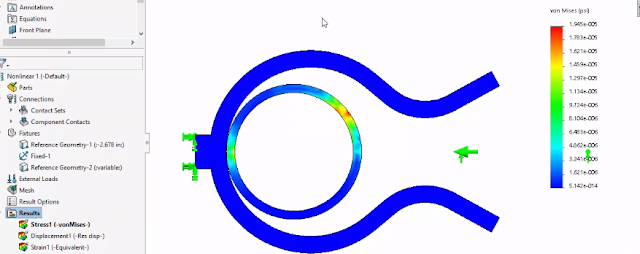Stress result, allow you to determine the stress level base on the different position. Picture above is the result after the ring moved into the hook.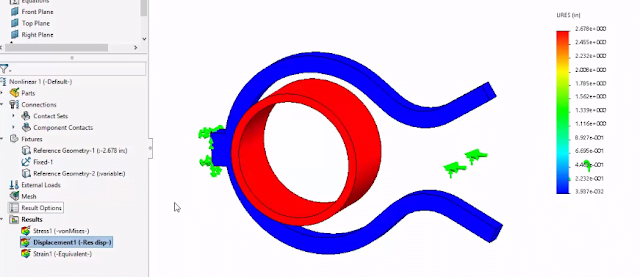Since the ring moved the most in the simulation, the ring remain in red colour which indicate the object moved the most distance in the simulation.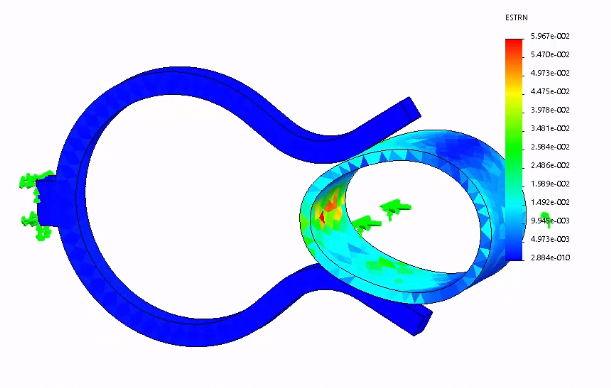Deformation when the ring moved into the hook.